﻿ Trilinear Interpolation Algorithm for Reconstruction of 3D MRI Brain ImageAmerican Journal of Signal Processing

p-ISSN: 2165-9354    e-ISSN: 2165-9362

2017;  7(1): 1-11

doi:10.5923/j.ajsp.20170701.01### Trilinear Interpolation Algorithm for Reconstruction of 3D MRI Brain Image

Cam Q. T. Thanh, Nguyen T. Hai

Faculty of Electrical and Electronics Engineering, HCMC University of Technology and Education, Ho Chi Minh City, Vietnam

Correspondence to: Nguyen T. Hai, Faculty of Electrical and Electronics Engineering, HCMC University of Technology and Education, Ho Chi Minh City, Vietnam.
 Email: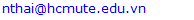Abstract

Medical image processing to support doctors for exact diagnosis and early treatment plays an important role. In this paper, construction of a three-dimensional (3D) image from 2DMRI cortex images is proposed. In addition, the 3D image may represent the visual details of brain inside human cortex. The 2DMRI cortex images are pre-processed using the mean filer for removing multiplicative noises and the histogram equalization for enhancement is applied. In addition, the multilevel Otsu method is employed to separate brain part inside the human cortex and a proposed algorithm of the trilinear interpolation is utilized for the construction of a 3D image. Simulation results show that the effectiveness of the proposed approach and also it is a sharing information for developments of 3D image construction.

Keywords: MRI brain image, 3D Construction, Multilevel Otsu and Region Growing, Trilinear interpolation

Cite this paper: Cam Q. T. Thanh, Nguyen T. Hai, Trilinear Interpolation Algorithm for Reconstruction of 3D MRI Brain Image, American Journal of Signal Processing, Vol. 7 No. 1, 2017, pp. 1-11. doi: 10.5923/j.ajsp.20170701.01.

### 1. Introduction

Medical image diagnosis plays an increasingly important role in modern medicine. In addition, detection and diagnosis of early potential risks causing cancer for treatment easier is necessary and less costly. In practice, there are many diagnostic imaging techniques such as Positron Emission Tomography (PET), Computed Tomography (CT) Scanner, Magnetic Resonance Imaging (MRI) [1-3].
There have been many researches about the process of building 3D images from 2D medical images in recent years. The 3D surface of the knee or a 3D spine image was constructed from 2D CT images [1, 2] using Marching Cube. This method allows to divide data blocks into cubes and each cube was made up of eight adjacent voxels. From these eight adjacent voxels, material surfaces were built using the triangular mesh. Therefore, the method has the advantage of fast calculation, simple construction operations and produces 3D images with the high resolution. However, the calculation will be slow, if one processes the large number of 2D image data. In addition, the images captured from sensors often have noise, because to improve the quality of constructing a 3D image, 2D images need to be pre-processed to reduce noise. In particular, a mean-unsharp filter may be applied to enhance high frequency components and filtered noise.
Enhancement method of MRI images  was employed by processing the intensity values of grayscale image before low-level image separation. Therefore, low-contrast images may be converted to images with higher contrast. Moreover, morphological operations are often used to make the tumor boundary. In this paper, morphological operations are utilized to allow to stretch and then fill the object for segmentation. The segmentation of image is an important step to build 3D image. Otsu method is often applied to find the gray level threshold values to segment 2D images for construction of 3D image [4, 5]. In this research, the Otsu algorithm will allow to determine an appropriate threshold for segmentation of 2D MRI images .
A segmentation method is often used in identifying tumors or in building 3D images. An algorithm of regional development was combined with the segmentation method based on the similarity of adjacent pixels with the nuclear point . The result of the combined algorithm depends very much on choosing where the nuclear initial point and the error between the nuclear point and neighboring pixels. In practice, the process of 2D medical image acquisition may appear noise on it. Therefore, a threshold of the traditional Otsu method may be employed to segment some areas for building 3D image. However, this method may lead to the poor result. For the better results, an Otsu method with multilevel are applied to divide 2D image into many layers.
Pixels in 2D image have the same regions combined with algorithm of region development  to segment the image. The 2D image after segmentation may be processed in the coordinate axes (x, y, z). In particular, linear interpolation algorithm is utilized to construct the 2D image surface by calculating the approximate value of a point between two consecutive layers in the spatial domain [9, 10].
This article has the main sections. In particular, Section-I introduces the necessity of building a 3D image based on 2D MRI images and some relevant techniques. In Section-II, the paper will present the image pre-processing problems such as image segmentation, region development for 3D construction. Section-III describes results and discussion. Finally, the conclusion is presented in Section-IV.

### 2. Materials and Methods

Dataset with 44 2D MRI brain images with 256x192 pixels, which are provided by Binh Duong General Hospital, are used for construction of 3D image in this research.
3D image construction from 2D MRI human cortex images will be performed the following steps as described in Figure 1. Firstly, the 2D MRI images are pre-processed, including noise rejection and image enhancement. The second is that the morphological operator is employed in order to remove pixels around boundaries of objects in the 2D images. Thus, the pre-processed images are segmented for the purpose of separating the brain area from the cortex for 3D construction. Finally, the 2D images after the segmentation will be constructed to produce the 3D image using a trilinear interpolation algorithm as shown in Figure 1.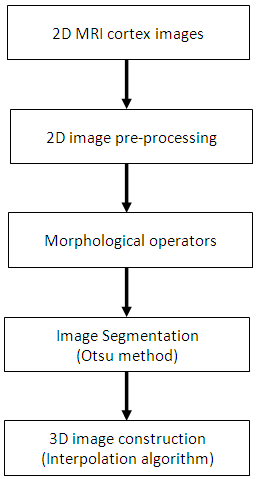Figure 1. Block diagram of 3D image construction

#### 2.1. Image Pre-processing

For constructing 3D image, 2D MRI images with noise are smoothed using an average filter. This average filter is convoluted with each 2D MRI image to remove noise using the following equation: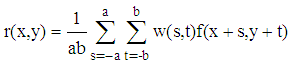(1)
in which w(s,t) is the axb filter window, and r(x,y) is the output image after the filter.
Figure 2 shows the result of a 2D MRI cortex image after convoluting to an average filter with a 3-by-3 kernel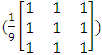. Thus, the convoluted image is processed to be smoother and it also retains the complete brain region after separating from the cortex image compared with the image using the median filter as shown in Figure 3.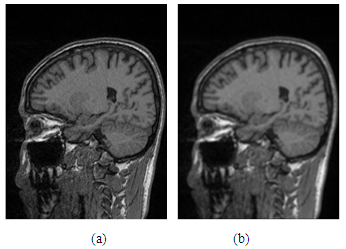Figure 2. (a)-Original human cortex image; (b)-Cortex image after the mean filter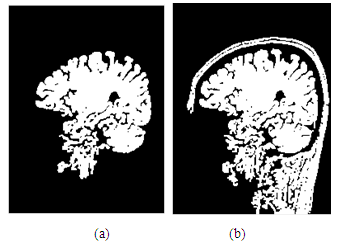Figure 3. (a)-image after using the mean filter; (b)-image after using the median filter
Moreover, a unsharp filter with the size of 3x3,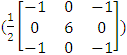is applied to remove the low frequency components and to enhance the image with the high frequency components as shown in Figure 4.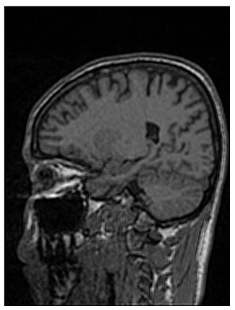Figure 4. 2D MRI cortex image after the unsharp filter
An image after filtering can has low contrast, in this case, the image needs to be enhanced to make objects on it better. A histogram equalization algorithm is employed to transform the image having higher contrast by spreading the pixel values in the whole image . The image enhancement using the histogram equalization is described using the following expression: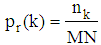(2)
in which pr(k) is the probability density function (PDF) with the kth gray level values in the image, r(x,y); MN is the total pixels of the image; nk denotes the number of pixels at the kth gray level in the input image.
From this PDF, the output expression of the image is used to calculate as follows: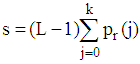(3)
in which s(x,y) is the number of the pixels at the kth gray level of the corresponding output image; L is the number of gray levels in the image.
Figure 5 and Figure 6 represent images before and after enhancement, in which Figure 6 shows the histogram graphs with gray level values. In particular, the unsharp image with high frequency components was enhanced using the histogram equalization to create higher contract. Figure 6(a) represents the large number of pixels distributed closed to the zero point, while the enhanced image in Figure 6(b) has the pixel values spreading on the gray level axis.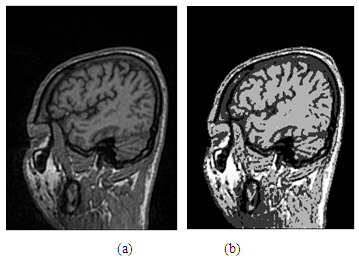Figure 5. (a)-Image using the unsharp filter; (b)-Image after enhancement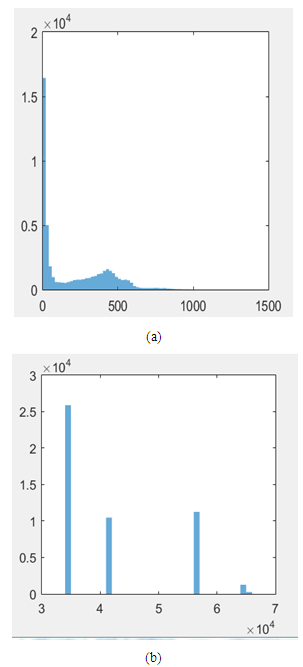Figure 6. Representation of images: (a)-Image before enhancement; (b)-Image after enhancement using the histogram equalization

#### 2.2. Morphological Operation

After filtering 2D MRI cortex image to remove noise and then enhancing it, the enhanced image is processed for imperfection of the image. Therefore, a morphological algorithm is applied to remove some parts which are not necessary around objects in the image. In particular, the image using the morphological algorithm just retains the important object for construction of 3D image. In particular, in the morphological operations including Dilation and Erosion, the dilation operator is employed to process the 2D enhanced image based on its shapes and structures. In this case, the morphological operation is used to combine boundaries of objects and to remove the undesired parts around the objects . For image calculation, the morphological image is performed by convoluting between the input image and a kernel as follows: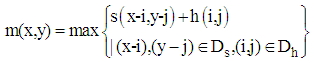(4)
where m(x,y) and s(x-i,y-j) are the input and output images and h(i,j) is a kernel with the size of 2x2 as shown in Figure 7, Ds and Dh are the domains of the image s and the kernel h.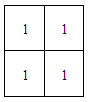Figure 7. Structuring matrix of the 2x2 kernel
From the enhanced image as shown in Figure 8(a), the morphological algorithm with the dilation operator was utilized to produce the 2D MRI cortex image with enlarging boundaries of regions of the object as shown in Figure 8(b). Therefore, Figure 8(b) shows that the boundaries of the object regions are combined and the morphological image is better compared to that of Figure 8(a).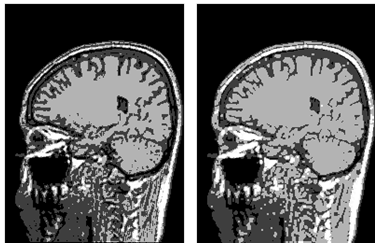Figure 8. (a)-Representation of the enhanced image; (b)-Image after the morphological operation

#### 2.3. Otsu Method for Image Segmentation

Image segmentation plays an important role in constructing a 3D medical image. In this research, all slices of 2D brain images are segmented before construction. An Otsu algorithm is employed for these 2D MRI image segmentation. In particular, this algorithm allows to calculate many gray level thresholds and the threshold value is chosen corresponding to the minimum variance within the class. The segmentation algorithm for the set of 2D MRI cortex images is expressed as flows: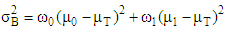(5)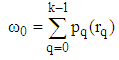(6)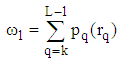(7)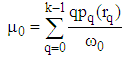(8)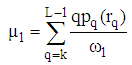(9)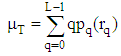(10)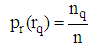(11)
in which n is the total number of pixels in the image, nq denotes the total number of pixels with gray level rq and L describes the maximum gray level of the image,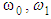are the background and foreground variances, pr(rq) describes the probability density function of the image histogram.
Figure 8 shows the result of the 2D cortex image after segmentation using the traditional Otsu method from the morphological image in Figure 8(b). In the segmentation using the traditional Otsu method with the 7 thresholding, the image has advantages of making the quick segmented image. However, the segmentation of this traditional method completely depends on a threshold based on the minimum variance, so the segmented image may make the undesired object with some lost parts as the blue circle shown in Figure 9.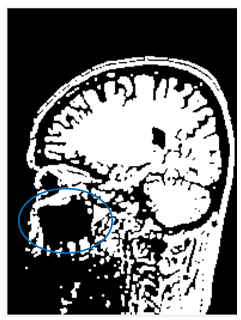Figure 9. MRI cortex image segmented using the traditional Otsu method with the 7 thresholding
As shown in Figure 8, the mouth signed blue in the segmented image using the traditional Otsu method is disappeared. In similarity, Figure 10 shows the result of the spine image after segmentation of the same Otsu method and some objects in this are disappeared .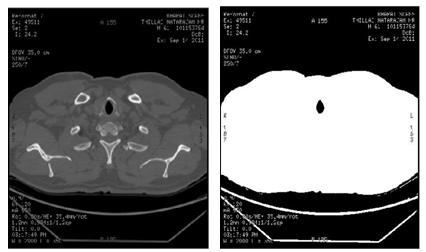Figure 10. (a)-Original image; (b)-image after the traditional Otsu segmentation
With using the traditional Otsu method, the result is obvious that choosing a threshold for the image segmentation is important related to 3D image construction. Because the segmentation to collect images with the desired objects will produce the better result of constructing the 3D image. This will enhance for diagnosis of doctors.
For improvement, an Otsu method with multilevel is utilized. In particular, the multilevel of this method are selected to retain the whole desired objects in the image and it will remove unnecessary parts before construction of 3D image . In this article, the Otsu segmentation method with two thresholding multilevel is proposed and its expression is described as follows: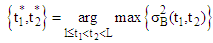(12)
in which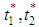are two thresholds chosen corresponding to the maximum variance within the class.
Figure 11 shows the simulation result of the segmented image using the traditional Otsu method with two thresholds, in which the mouth object in this image is seen more clearly compared to that in Figure 9.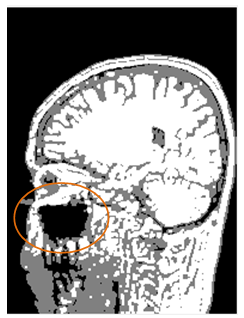Figure 11. Representation of the 2D MRI cortex segmented using the Otsu method with two multilevel
The segmented image using the Otsu multilevel method is developed in the region. In particular, the 2D MRI cortex image after segmentation is separated a desired region for construction of 3D image, in which the algorithm of growing region is utilized from a point which is called the seed in the image. Therefore, the area will be expanded to neighboring pixels according to some criteria. In this technique, the image is grouped with adjacent pixels to form a region and the combination of pixels is performed based on common standards.
Assume that m(x,y) is the pixel intensity of the image at coordinates (x, y). Thus a pixel nay be added to a group, if it meets the absolute difference value compared to the intensity of the central point, m(xseed,yseed). The difference value, α is described as in the following inequality: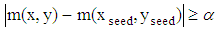(13)
With the region growing algorithm, one will select of the initial point between neighboring pixels to the central point so that this initial point will produce the difference. As shown in Figure 12 and Figure 13, the selection of the initial point (point-1 or point-2) may produce the difference value and they are represented as in Figure 12(b) và Figure 13(b) .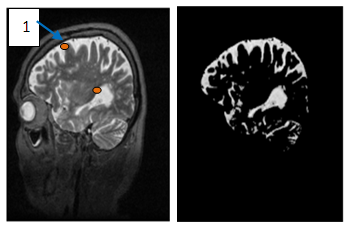Figure 12. (a)-Enhanced image, (b)-Brain object after segmentation combined with the region growing corresponding to the seed positioned at point-1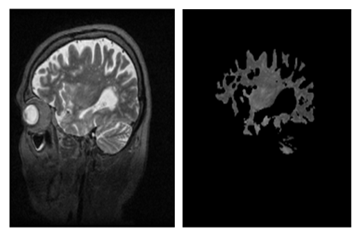Figure 13. (a)-Enhanced image, (b)-Brain object after segmentation combined with the region growing corresponding to the seed positioned at point-2
Therefore, the Otsu segmentation method based on two thresholds combined with the region growing was employed were applied to produce the binary image. This image has the black areas corresponding to the gray level values, 1 and 2, while the gray level value, 3 is white. This means that the region in the image has the uniform gray level value, so the selection of a regional central point will not affect the output results.
As the results shown in Figure 14, one may see the original location of the different center and the image after segmentation has no change much. For reconstruction of 3D image, the segmented image is multiplied with the original image to obtain a characteristic image including the desired objects. In particular, each pixel in the original image r(x,y) is multiplied by the corresponding pixel in the segmented image m(x,y) to produce the desired image C(x,y) with the brain object and its completely black background. In particular, the original image as described in Figure 2 is multiplied the segmented image as shown in Figure 14(c) to produce the multiplied image, just being the brain object in the 2D MRI cortex image as shown in Figure 15.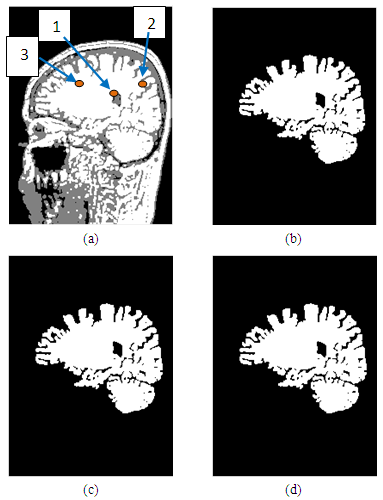Figure 14. Representation of the processed images, in which (a)-the enhanced image; (b),(c),(d)-Images after segmentation with seed positioned at points 1, 2, 3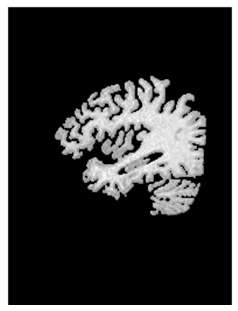Figure 15. Image representation after convolution between the original and the segmented image

#### 2.4. Interpolation Algorithm for 3D Construction

A constructed 3D brain image consists of a set of many 2D images, there are 44 image slices in this research. 2D brain images after segmentation are built to overlap in order on a coordinate system (x,y,z) [15-20]. In this paper, the 3D brain image is not only constructed based on 44 2D brain images to view the brain object surface, but also it is constructed to observe problems inside this 3D brain part at different angles. A trilinear interpolation method is employed to calculate pixels between two slices as modelled in Figure 16.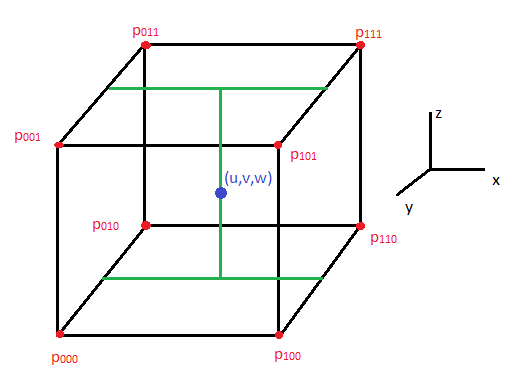Figure 16. Representation of a trilinear interpolation model
The trilinear interpolation method is used to construct a typical object from a set of discrete points. In this method, the value of an approximate point of the 2D images in the spatial domain (u, v, w) as shown in the rectangular box of Figure 15 is calculated using the following equation: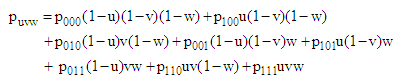(14)
in which puvw is the intensity of the pixels at coordinates (u, v, w).

### 3. Results and Discussions

In this research, a set of 44 MRI cortex images were collected from an MRI machine in Binh Duong General Hospital. The simulation results show that in order to construct a 3D brain image, 2D MRI cortex images need to be processed for enhancement and segmentation of these 2D images as shown in Figure 17 and Figure 18. In the image segmentation, the thresholds with two levels were calculated to produce the desired image.
The image is segmented by the Otsu multilevel method as shown in Figure 17(d). The segmented images are continued to process using the region growing method as described in Figure 17(e). It is obvious that the segmented image is the binary image with the pixel values, [0 1]. This 2D image will be used to construct a 3D image with the desired object.
Figure 18 shows 12 original MRI cortex images composing of slices 3, 6, 13, 17, 19, 22, 24, 26, 28, 31, 34, 44 of 44 images.
Figure 18 represents the simulation results of the segmented images to show the brain objects using the Otsu method with two thresholds combined with the region growing algorithm from 12 MRI cortex images in Figure 17.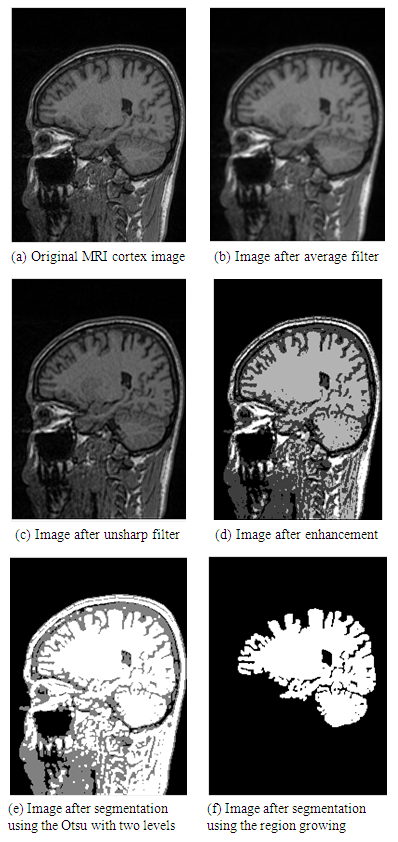Figure 17. Representation of the pre-processing image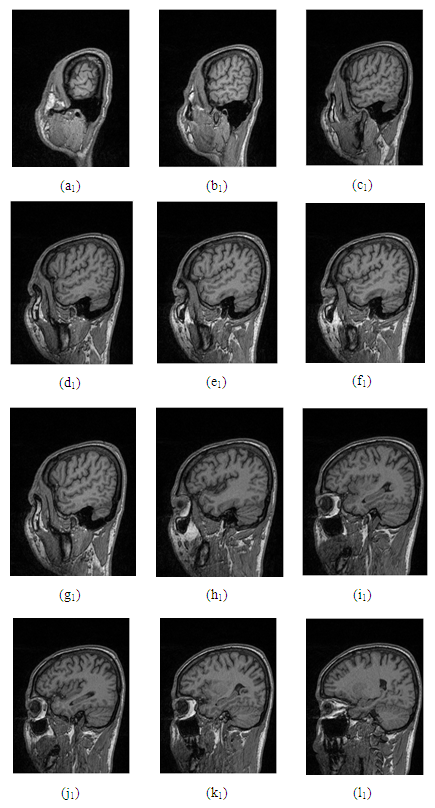Figure 18. Representation of the morphological cortex images
The segmented brain images obtained are the binary images which retain just the brain part in the whole MRI cortex image. The binary image after processing to produce the 3D image having the structure of the original image.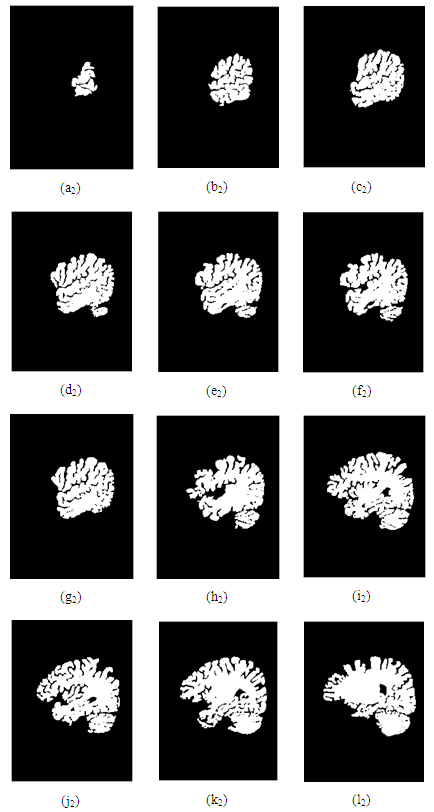Figure 19. 12 brain images after segmentation
Figure 20 represents the convoluted images between the segmented image and the corresponding original images of 12 image slices of 44 2D MRI cortex images. From these 2D convoluted images with the brain objects, one was constructed the 3D image displayed the brain object as shown in Figure 21 using the trilinear interpolation method.
Therefore, the 3D images are synthesized in order at coordinate (x,y,z) and interpolate between two image slices of consecutive points using the trilinear interpolation method. Moreover, Figure 21 represents the 3D brain images which are rotated to consider at angles of 45°, 90° and other positions depending on viewing.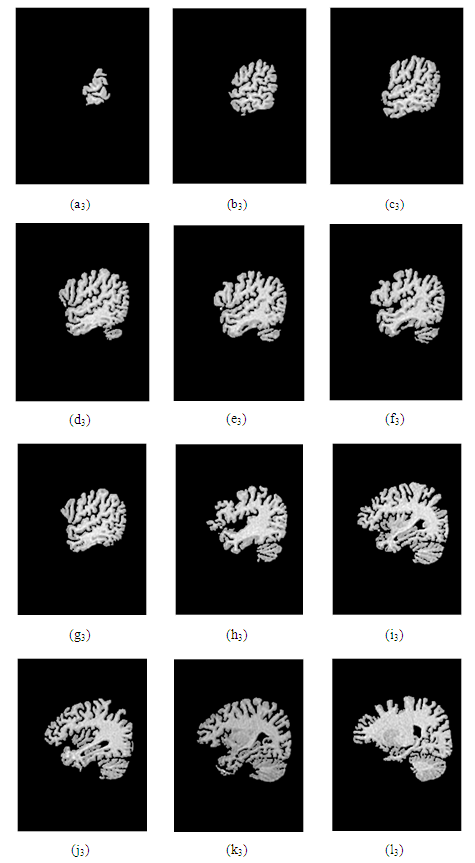Figure 20. Images after convolution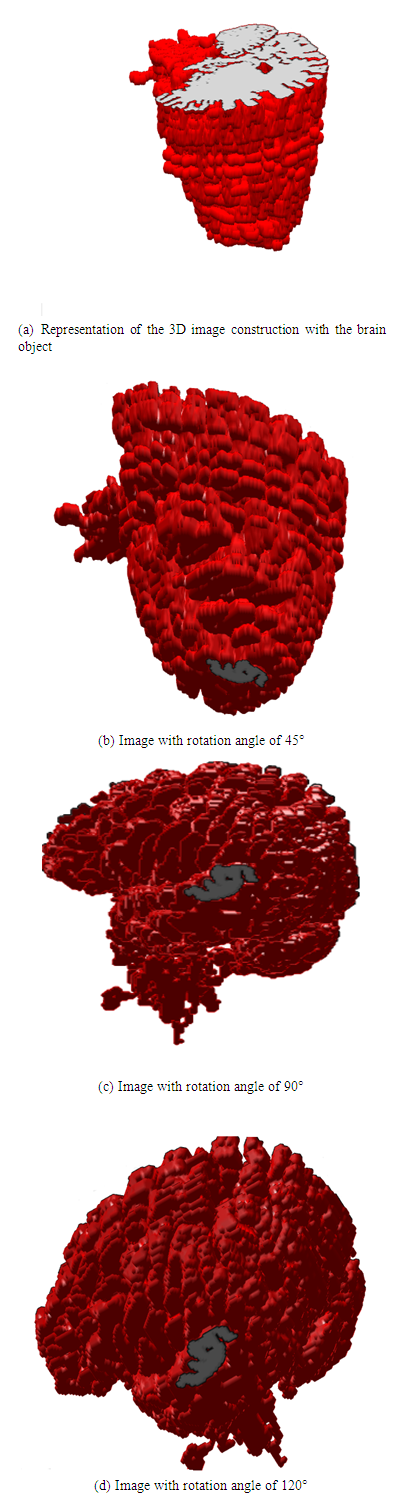Figure 21. The 3D model of brain viewed from different elevations and azimuth angles
Figure 22 shows the result after the 3D construction of the 2D human knee CT images using a marching cube method . This method has the advantage of quickly constructing the surface with different elevations and azimuth angles. However, its disadvantage is that these 3D images may be observed only outside the knee images. While, observation of problems inside the 3D images for exact diagnosis is necessary, because doctors may obtain more information around the knee. The main weakness of the marching cube method is that one couldn’t view objects/regions inside the reconstructed 3D image.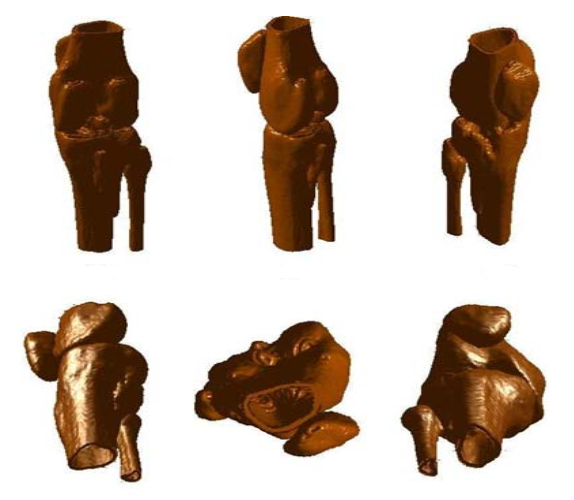Figure 22. 3D models of the knee image viewing from different elevations and azimuth angles 
In this paper, the proposed method for the reconstruction of the 3D MRI brain image to replace for the marching cube is the trilinear interpolation. This method shows not only view of the brain surface at different angles, but also allows designing to rotate the 3D image into the desired position for observing objects/regions inside the reconstructed 3D brain image. However, the view in detail depends on the large number of 2D medical images and the selection of thresholds for image segmentation. The simulation results effectively showed details at the surface and objects inside the constructed 3D image for observation and diagnosis.
In this reseach, 3D reconstruted images using the trilinear interpolation method show the image with other shapes which are processed by cutting the brain part to be able to view other problems inside the brain as described in Figure 23(a). In Figure 23(b) to Figure 239d), the 3D images after cutting are rotated at different angles for viewing their inside problems. In addition, Figures 23(e) and (f) represent the 3D images constructed and rotating from four brain slices for observing at other positions of the brain image.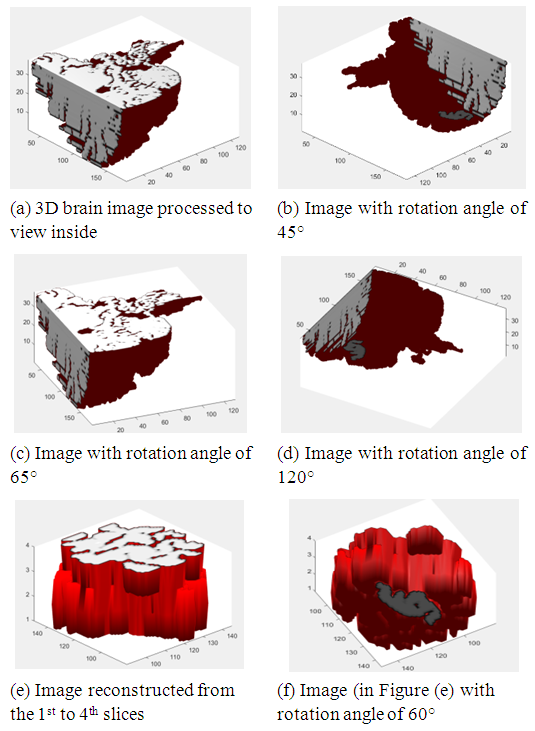Figure 23. Representation of the 3D reconstructed image with cutting slices and rotating them

### 4. Conclusions

In this paper, 44 2D MRI cortex images were pre-processed before construction of a 3D brain image. In particular, the brain parts in the 2D cortex images which need to observe at different angles, are separated. From the 2D images, after enhancement of the images using the histogram equalization, the multilevel Otsu method was applied to segment each human cortex image to produce the part of brain for consideration and diagnosis. For observation at different angles of the 3D brain image, the trilinear interpolation method was utilized to construct the 3D brain image from the enhanced 2D MRI images. Simulation results shows that the 3D brain image which allows to view at different angles may support for doctors in early diagnosing problems inside human brain cortex for treatment easier.

### ACKNOWLEDMENTS

The author is highly grateful to Binh Duong General Hospital, where provided MRI image database for this research.

### References

  J. Athertya and S. Poonguzhali, "3D CT Image Reconstruction of the Vertebral Column", International Conference on Recent Trends in Information Technology, pp. 81 - 84, 2012.  Avnish Patel and Kinjal Mehta, "3D Modeling and Rendering of 2D Medical Image", International Conference on Communication Systems and Network Technologies, pp. 149 - 152, 2012.  Senthilkumaran N and Thimmiaraja, "Histogram Equalization for Image Enhancement Using MRI brain images", WCCCT, pp. 80 - 83, 2014.  M. S. Sindhuri and N. Anusha, "Text Separation in Document Images through Otsu’s Method ", International Conference on Wireless Communications, pp. 2395 - 2399, 2016.  Mengxing Huang, Wenjiao Yu and Donghai Zhu, "An Improved Image Segmentation Algorithm Based on the Otsu Method", International Conference on Software Engineering, Artificial Intelligence, Networking and Parallel, pp. 135 - 139, 2012.  C. Zhou, Liwei Tian, Hongwei Zhao and Kai Zhao, "A method of Two-Dimensional Otsu image threshold segmentation based on improved Firefly Algorithm", International Conference on Cyber Technology in Automation, Control, and Interlligent systems, pp. 135 - 139, 2012.  Y. Ho, W. Lin, C. Tsai, C. Lee and Chih Y. Lin, "Automatic Brain Extraction for T1 – Weighted Magnetic Resonance Images Using Region Growing", International Conference on Bioinformatics and Bioengineering, pp. 250 - 253, 2016.  X. Zhang, X. Li, H. Li and Yuncong Feng, "A Semi – Automatic Brain Tumor Segmentation Algorithm", International Conference on Multimedia and Expo, pp. 1 - 6, 2016.  Robert Hagan, "Numerical Methods for Isosurface Volume Rendering", Virginia Polytechnic, 2009.  P. Stelldinger, L. J. Latecki and M. Siqueira, "Topological Equivalence between a 3D Object and the Reconstruction of Its Digital Image", IEEE Transactions on Pattern Analysis and Machine Intelligence, pp. 126 - 140, 2007.  Hardeep Kaur and J. Rani, "MRI brain image enhancement using Histogram equalization Techniques", International Conference on Wireless Communications, Signal Processing and Networking, pp. 770 - 773, 2016.  M. Sahar, H. A. Nugroho, Tianur, I. Ardiyanto and L. Choridah, "Automated Detection of Breast Cancer Lesions Using Adaptive Thresholding and Morphological Operation", International Conference on Information Technology Systems and Innovation, pp. 1 - 4, 2016.  X. He, Zhenkuan Pan, Qian Dong and G. Wang, "Veins Segmentation and Three-Dimensional Reconstruction from Liver CT Images Using Multilevel OTSU Method", International Conference on Image and Graphics, pp. 248 - 251, 2013.  Lei Guo, Ming Hu, Ying Li, Weili Yan and L. Zhao, "Three Dimension Reconstruction of Medical Images Based on an Improved Marching Cubes Algorithm", International Conference on Biomedical Engineering and Informatics, pp. 64 - 68, 2013.  A. Nachour, L. Ouzizi and Y. Aoura, "Multi-Agent 3D Reconstruction of Human Femur from MR Images", International Conference on Intelligent Systems Design and Applications, pp. 88 - 92, 2015.  Roshnara Nasrin and S. Jabbar, "Efficient 3D visual hull reconstruction based on marching cube algorithm", International Conference on Innovations in Information, Embedded and Communication Systems, pp. 1 - 6, 2015.  F. Paulano, J. J. Jimenez and R. Pulido, "An application to interact with 3D models reconstructed from medical images", International Conference on Computer Vision Theory and Applications, pp. 224 -229, 2014.  Hongjian Wang, "Three-Dimensional Medical CT Image Reconstruction", International Conference on Measuring Technology and Mechatronics Automation, pp. 548 - 551, 2009.  V. S. Nguyen, M. H. Tran and M. Q. Vu, "A Research on 3D Model Construction from 2D DICOM", International Conference on Advanced Computing and Applications, pp. 158 - 163, 2016.  Chung. S. Wang, Man - Ching Lin, C. C. Wang, Ching-Fu Chen and Jei-Chen Hsieh, "Feature reconstruction for 3D medical images processing", International Conference on Biomedical Engineering and Informatics, pp. 69 - 74, 2013.